Pages 63 to 85

Index
Pages:    1 to 21
Pages:    22 to 41
Pages:    42 to 62

The pages can be printed and collated into a book for easy reference.

More circuits and projects can be found on TALKING ELECTRONICS website:
Colin Mitchell
talking@tpg.com.au

Tel: 0417 329 788

 CONTENTS AC Trigger    Ammeter   Amplifier   Arc Welder Audio Alarm   Audio Mixer BC 547    NPN transistor BC557     PNP transistor BD 139   BD140 transistor Bipolar Transistors   CA 3130 OP-amp Capacitor Data Car Voltage Converter  Chip Pinouts Circuit Symbols  Common Base/Emitter/ Collector Circuits  Counter  Crystal Set    Darlington Transistor   DC Millivoltmeter   Definitions    Difference Amplifier Diode  Dual Power Supply Electronic Dice    FET    FET Voltmeter   Field Strength Meter MkII Flashing LED   FM Transmitter    FM Bug Germanium Diode  Hearing Aid Infinity Bug Infrared LED Infrared Light Beam  Kitt Scanner Lamp Dimmer    Lamp Flasher  Light Beam Relay   Light Switch   LEDs   LED Chaser LED Dice LED Flasher LM 340   LM 386 Logic Gates Logic Probe   Metal Detector  Metronome   Microcontrollers 39 83 20, 21 78 59 46 19 19 21 15 52 71 25 74 4 15,16 37 9 17 47 18 46 8 54 36 29 29 79 33 20,81 9 81 80 34 38 60 39 59 28 30 31 33 61 33 50 67 56 58,62 57,59 25 85 Microphone  Morse Code Generator MPF 102  FET Multivibrator   OA 91  diode  OP Amp   Peak Reading VU Meter Photo Diode   Photo Electric Relay  Pinouts Power Diode   Power Supply Relay Driver Resistors   RF Monitor Meter   RF Prescaler  SCR   C122D Silicon Controlled Rectifier  Simon Regulator 78xx  79xx Signal Diode    Semiconductor Devices   Solar Charger Square Wave Oscillator Steam Simulator Surface Mount Resistors Time Delay   Timer Transistor Amplifier Transistor Pinouts  TRIAC   SC151D Touch Switch    Ultrasonic Transmitter Unijunction Transistor  UJT   UJT Time Delay  Wein Bridge Oscillator   Zener Diode    1N4001   Power diode 1N4148  Signal diode 27MHz Links 2N2646  UJT 2N 3055   transistor 4017   Decade counter 555 Light Switch    555 Timer   7-Segment Displays 74c14 Hex Schmitt  741   OP Amp 7555   CMOS 555 7805   +5v  Regulator 7905   -5v  Regulator 83 21 26 21 9 42 52 34 30 74 8 22, 60 20 68 9 28 40 44 86 8, 10 51,53 14 56 77 50 75 69 25 28 81 55 38 58 57 37 37 53 47 8 10 23 76 22 64 60 29 48 35 62 44 50 60

Page 63

40106 OR 74C14 HEX Schmitt Trigger IC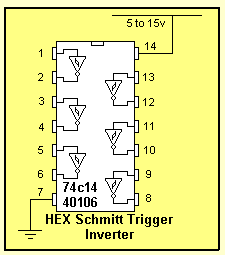This chip is known by a number of identities. 74C14. It is also marketed as 40106, 40014, and 74HC14.  These are all CMOS chips and are characterised by low current consumption, high input impedance and a supply voltage from 5v to 15v. (Do not substitute 7414 or  74LS14. They are TTL chips and operate on 4.5v to 5.5v and have low impedance inputs.) The 74C14 contains 6 Schmitt Trigger gates. Minimum supply voltage 5v Maximum supply voltage 15v Max current per output 10mA Maximum speed of operation 4MHz Current consumption approx 1uA with nothing connected to the inputs or outputs.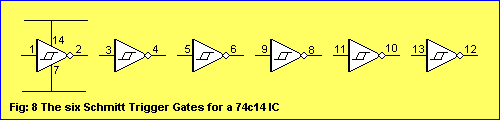Here are some of the things you can do with the gates in the 40106 Hex Schmitt Trigger chip:

INVERTING
If the output is required to be the opposite of the circuit above, an inverter is added: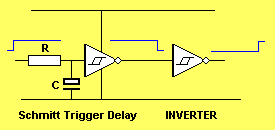If a diode is added across the input resistor, the capacitor "C" will be discharged when the input goes low, so the "Delay Time" will be instantly available when the input goes HIGH: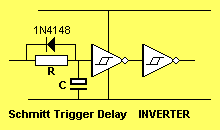The following circuit produces a PULSE (a LOW pulse) when the input goes HIGH: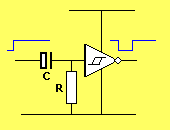Page 64

To invert the output, add an inverter: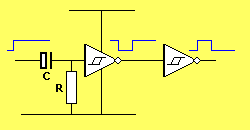To produce a pulse after a delay, the following circuit can be used: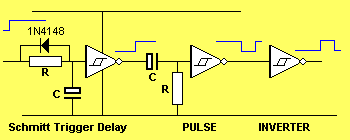The following circuit produces a tone during the HIGH period. When the output of the second inverter is HIGH, it places a high on the input of the third inverter, via the diode. This is called "jamming" the oscillator and prevents the oscillator from operating. When the second inverter goes LOW, the oscillator will operate.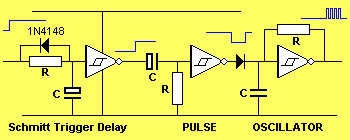The oscillator above can be set to produce a 100Hz tone and this can activate a 2kHz oscillator to produce a 2-tone output. A "jamming diode" is needed between the third and fourth gates to allow the high-frequency oscillator to operate when the output of the low-frequency oscillator is HIGH.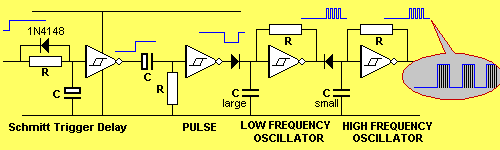The output can be buffered with a transistor: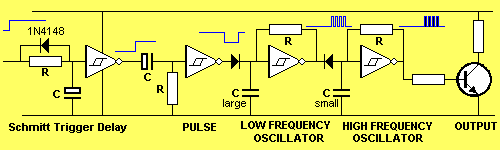Page 65
Extending the action of a push button

The action of a push button can be extended by adding the following circuit: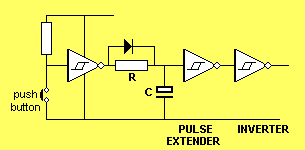To produce a pulse of constant length, (no matter how long the button is pressed), the following circuit is needed: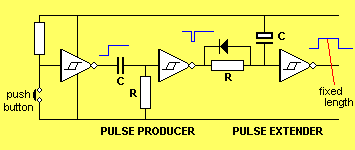GATING
Gating is the action of preventing or allowing a signal to pass though a circuit.
In the following circuit, buttons "A" and "B" are gated to allow the oscillator to produce an output.
The first two inverters form an "OR-gate." When the output of the gate is HIGH it allows the oscillator to operate.The second diode is called the gating diode. When the output of the second inverter is LOW, the capacitor is prevented from charging as the diode will not allow it to charge higher than 0.7v, and thus the oscillator does not operate.
When the output of the second inverter is HIGH, the capacitor is allowed to charge and discharge and thus oscillator will produce an output. If the push buttons can be placed together, the circuit can be simplified to:PULSER
The 74c14 can be used to produce a 3mS pulses every second. The circuit is adjustable to a wide range of requirements.Page 66

2 MINUTE TIMER
Some of the features we have discussed have been incorporated into the following circuit. The relay is energized for a short time, 2 minutes after the push-button is pressed. The push-button produces a brief LOW on pin 1, no matter how long it is pushed and this produces a pulse of constant length via the three components between pin 2 and 3.
This pulse is long enough to fully discharge the 100u timing electrolytic on pin 5.
The 100k and electrolytic between pins 6 and 9 are designed to produce a brief pulse to energize the relay.TRIGGER TIMER

The next design interfaces a "Normally Open" and "Normally Closed" switch to a delay circuit.
The feedback diode from the output prevents the inputs re-triggering the timer (during the delay period) so that a device such as a motor, globe or voice chip can be activated for a set period of time.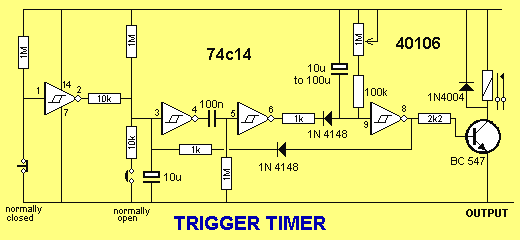ALARM
In the following circuit, the gates are used to detect the touch of a door knob and produce an output that goes HIGH for approx 1 minute.The output of the above circuit can be taken to an alarm. Open the reed switch contacts and connect the reed switch to the output of the Door-knob alarm.Page 67

LM 386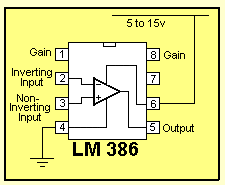300mW amplifier using LM 386300mW amplifier using LM 386

Page 68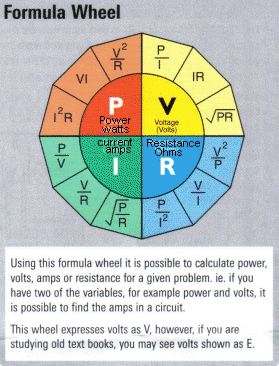Page 69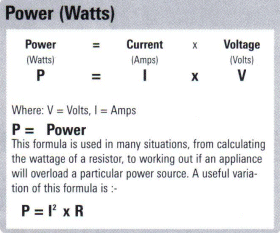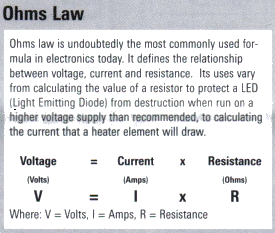A 330k SM resistorSurface Mount Resistors
All SM resistors conform to a 3-digit or 4-digit code. But there are a number of codes, according to the tolerance of the resistor. It's getting very complicated.
Here is a basic 3-digit SM resistor:

The first two digits represent the two digits in the answer. The third digit represents the number of zero's you must place after the two digits. The answer will be OHMS. For example: 334 is written 33 0 000. This is written 330,000 ohms. The comma can be replaced by the letter "k". The final answer is: 330k.
222 = 22 00 = 2,200 = 2k2
473 = 47 000  = 47,000 = 47k
105 = 10 00000 = 1,000,000 = 1M = one million ohms
There is one trick you have to remember. Resistances less than 100 ohms are written: 100, 220, 470. These are 10 and NO zero's = 10 ohms = 10R
or 22 and no zero's = 22R  or 47 and no zero's = 47R.  Sometimes the resistor is marked: 10, 22 and 47 to prevent a mistake.
Remember:
R = ohms     k = kilo ohms = 1,000 ohms     M = Meg = 1,000,000 ohms
The 3 letters (R, k and M) are put in place of the decimal point. This way you cannot make a mistake when reading a value of resistance.

THE COMPLETE RANGE OF SM RESISTOR MARKINGS:

 0R1 = 0.1ohm R22 = 0.22ohm R33 = 0.33ohm R47 = 0.47ohm R68 = 0.68ohm R82 = 0.82ohm 1R0 = 1R 1R2 = 1R2 2R2 = 2R2 3R3 = 3R3 4R7 = 4R7 5R6 = 5R6 6R8 = 6R8 8R2 = 8R2 100 = 10R 120 = 12R 150 = 15R 180 = 18R 220 = 22R 270 = 27R 330 = 33R 390 = 39R 470 = 47R 560 = 56R 680 = 68R 820 = 82R 101 = 100R 121 = 120R 151 = 150R 181 = 180R 221 = 220R 271 = 270R 331 = 330R 391 = 390R 471 = 470R 561 = 560R 681 = 680R 821 = 820R 102 = 1k0 122 = 1k2 152 = 1k5 182 = 1k8 222 = 2k2 272 = 2k7 332 = 3k3 392 = 3k9 472 = 4k7 562 = 5k6 682 = 6k8 822 = 8k2 103 = 10k 123 = 12k 153 = 15k 183 = 18k 223 = 22k 273 = 27k 333 = 33k 393 = 39k 473 = 47k 563 = 56k 683 = 68k 823 = 82k 104 = 100k 124 = 120k 154 = 150k 184 = 180k 224 = 220k 274 = 270k 334 = 330k 394 = 390k 474 = 470k 564 = 560k 684 = 680k 824 = 820k 105 = 1M0 125 = 1M2 155 = 1M5 185 = 1M8 225 = 2M2 275 = 2M7 335 = 3M3 395 = 3M9 475 = 4M7 565 = 5M6 685 = 6M8 825 = 8M2 106 = 10M0

Page 70
The complete range of SM resistor markings for 4-digit code

0000 is a value on a surface-mount resistor. It is a zero-ohm LINK!
Resistances less than 10 ohms have  'R' to indicate the position of the decimal point.  Here are some examples:

 0000 =00R 00R1 = 0.1ohm 0R22 = 0.22ohm 0R47 = 0.47ohm 0R68 = 0.68ohm 0R82 = 0.68ohm 1R00 = 1ohm 1R20 = 1R2 2R20 = 2R2 3R30 = 3R3 6R80 = 6R8 8R20 = 8R2 10R0 = 10R 11R0 = 11R 12R0 = 12R 13R0 = 13R 15R0 = 15R 16R0 = 16R 18R0 = 18R 20R0 = 20R 22R0 = 22R 24R0 = 24R 27R0 = 27R 30R0 = 30R 33R0 = 33R 36R0 = 36R 39R0 = 39R 43R0 = 43R 47R0 = 47R 51R0 = 51R 56R0 = 56R 62R0 = 62R 68R0 = 68R 75R0 = 75R 82R0 = 82R 91R0 = 91R 1000 = 100R 1100 = 110R 1200 = 120R 1300 = 130R 1500 = 150R 1600 = 160R 1800 = 180R 2000 = 200R 2200 = 220R 2400 = 240R 2700 = 270R 3000 = 300R 3300 = 330R 3600 = 360R 3900 = 390R 4300 = 430R 4700 = 470R 5100 = 510R 5600 = 560R 6200 = 620R 6800 = 680R 7500 = 750R 8200 = 820R 9100 = 910R 1001 = 1k0 1101 = 1k1 1201 = 1k2 1301 = 1k3 1501 = 1k5 1601 = 1k6 1801 = 1k8 2001 = 2k0 2201 = 2k2 2401 = 2k4 2701 = 2k7 3001 = 3k0 3301 = 3k3 3601 = 3k6 3901 = 3k9 4301 = 4k3 4701 = 4k7 5101 = 5k1 5601 = 5k6 6201 = 6k2 6801 = 6k8 7501 = 7k5 8201 = 8k2 9101 = 9k1 1002 = 10k 1102 = 11k 1202 = 12k 1302 = 13k 1502 = 15k 1602 = 16k 1802 = 18k 2002 = 20k 2202 = 22k 2402 = 24k 2702 = 27k 3002 = 30k 3302 = 33k 3602 = 36k 3902 = 39k 4302 = 43k 4702 = 47k 5102 = 51k 5602 = 56k 6202 = 62k 6802 = 68k 7502 = 75k 8202 = 82k 9102 = 91k 1003 = 100k 1103 = 110k 1203 = 120k 1303 = 130k 1503 = 150k 1603 = 160k 1803 = 180k 2003 = 200k 2203 = 220k 2403 = 240k 2703 = 270k 3003 = 300k 3303 = 330k 3603 = 360k 3903 = 390k 4303 = 430k 4703 = 470k 5103 = 510k 5603 = 560k 6303 = 620k 6803 = 680k 7503 = 750k 8203 = 820k 9103 = 910k 1004 = 1M 1104 = 1M1 1204 = 1M2 1304 = 1M3 1504 = 1M5 1604 = 1M6 1804 = 1M8 2004 = 2M0 2204 = 2M2 2404 = 2M4 2704 = 2M7 3004 = 3M0 3304 = 3M3 3604 = 3M6 3904 = 3M9 4304 = 4M3 4704 = 4M7 5104 = 5M1 5604 = 5M6 6204 = 6M2 6804 = 6M8 7504 = 7M5 8204 = 8M2 9104 = 9M1 1005 = 10M

 Three Digit Examples Four Digit Examples 330 is 33 ohms - not 330 ohms 1000 is 100 ohms - not 1000 ohms 221 is 220 ohms 4992 is 49 900 ohms, or 49k9 683 is 68 000 ohms, or 68k 1623 is 162 000 ohms, or 162k 105 is 1 000 000 ohms, or 1M 0R56 or R56 is 0.56 ohms 8R2 is 8.2 ohms

A new coding system has appeared on 1% types. This is known as the EIA-96 marking method. It consists of a three-character code. The first two digits signify the 3 significant digits of the resistor value, using the lookup table below. The third character - a letter - signifies the multiplier.

 code value code value code value code value code value code value 01 100 17 147 33 215 49 316 65 464 81 681 02 102 18 150 34 221 50 324 66 475 82 698 03 105 19 154 35 226 51 332 67 487 83 715 04 107 20 158 36 232 52 340 68 499 84 732 05 110 21 162 37 237 53 348 69 511 85 750 06 113 22 165 38 243 54 357 70 523 86 768 07 115 23 169 39 249 55 365 71 536 87 787 08 118 24 174 40 255 56 374 72 549 88 806 09 121 25 178 41 261 57 383 73 562 89 825 10 124 26 182 42 237 58 392 74 576 90 845 11 127 27 187 43 274 59 402 75 590 91 866 12 130 28 191 44 280 60 412 76 604 92 887 13 133 29 196 45 287 61 422 77 619 93 909 14 137 30 200 46 294 62 432 78 634 94 931 15 140 31 205 47 301 63 442 79 649 95 953 16 143 32 210 48 309 64 453 80 665 96 976

Page 71

The multiplier letters are as follows:

 letter mult letter mult F 100000 B 10 E 10000 A 1 D 1000 X or S 0.1 C 100 Y or R 0.01

22A is a 165 ohm resistor, 68C is a 49900 ohm (49k9) and 43E a 2740000 (2M74). This marking scheme applies to 1% resistors only.

A similar arrangement can be used for 2% and 5% tolerance types. The multiplier letters are identical to 1% ones, but occur before the number code and the following code is used:

 2% 5% code value code value code value code value 01 100 13 330 25 100 37 330 02 110 14 360 26 110 38 360 03 120 15 390 27 120 39 390 04 130 16 430 28 130 40 430 05 150 17 470 29 150 41 470 06 160 18 510 30 160 42 510 07 180 19 560 31 180 43 560 08 200 20 620 32 200 44 620 09 220 21 680 33 220 45 680 10 240 22 750 34 240 46 750 11 270 23 820 35 270 47 820 12 300 24 910 36 300 48 910

With this arrangement, C31 is 5%, 18000 ohm (18k), and D18 is 510000 ohms (510k) 2% tolerance.
Always check with an ohm-meter (a multimeter) to make sure.

Chip resistors come in the following styles and ratings:
Style:
0402, 0603, 0805, 1206, 1210, 2010, 2512, 3616, 4022

Power Rating: 0402(1/16W), 0603(1/10W), 0805(1/8W), 1206(1/4W), 1210(1/3W), 2010(3/4W), 2512(1W), 3616(2W), 4022(3W)

Tolerance: 0.1%, 0.5%, 1%, 5%

Temperature Coefficient: 25ppm 50ppm 100ppm

CAPACITOR DATAA capacitor works on the principle of having two conductive plates which are very close and are parallel to each other. When a charge is applied to one plate of the capacitor, the electrons will generate an approximately equal, but opposite charge on the other plate. Capacitors will pass AC current, but will block DC current. A capacitor can also he used to smooth voltage ripple, as in DC power supplies.  Capacitance is measured in Farads (F). Capacitor Parameters Capacitors have five parameters: Capacitance (Farads), Tolerance (%), Maximum Working Voltage (Volts) Surge Voltage (Volts) and Leakage Because a Farad is a very large unit, most capacitors are normally measured in the ranges of pico, nano and micro farads. Working Voltage This refers to the maximum voltage that should be placed across the capacitor under normal operating conditions. Surge Voltage The maximum instantaneous voltage a capacitor can withstand. If the surge voltage is exceeded over too long a period there is a very good chance that the capacitor will be destroyed by the voltage punching through the insulating material inside the casing of the capacitor. If a circuit has a surging characteristic, choose a capacitor with a high rated surge voltage. Leakage Refers to the amount of charge that is lost when the capacitor has a voltage across its terminals. If a capacitor has a low leakage it means very little power is lost. Generally leakage is very small and is not normally a consideration for general purpose circuits. Tolerance As with resistors, tolerance indicates how close the capacitor is to its noted value. These are normally written on the larger capacitors and encoded on the small ones. Code      Tolerance                     Code       Tolerance C                ±.25pF                       D               ±0.5pF E                ±1pF                          G               ±2% J                ±5%                            K               ±10% L               ±15%                           M               ±20% N               ±30%                           Z               +80-20%   Capacitor Markings There are two methods for marking capacitor values. One is to write the information numerically directly onto the capacitor itself.  The second is to use the EIA coding system.   EIA Coding The EIA code works on a very similar principle to the resistor colour code.  The first two digits refer to the value with the third being the multiplier. The fourth character represents the tolerance. When the EIA code is used, the value will always be in Pico-Farads (see Decimal Multipliers). Example   103K This expands to: 1 =    1 0 =    0 3 =    x 1,000 K =    10% (sec Capacitor Tolerance for listings) Then we combine these numbers together: 1  0  x 1 000 =  10 000pF = 0.01µF,  =  10n    ±10% tolerance   Example 335K This expands to: 3 = 3 3 = 3 E = x100,000 K = ±10% Then we combine these numbers together 3 3 x100,000 = 3,300,000pF = 3,300nF = 3.3uF    10% tolerance.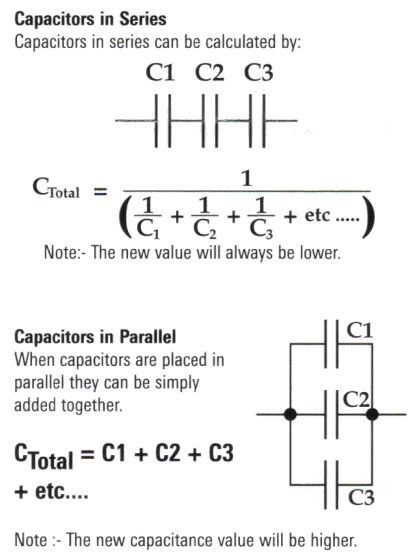Page 74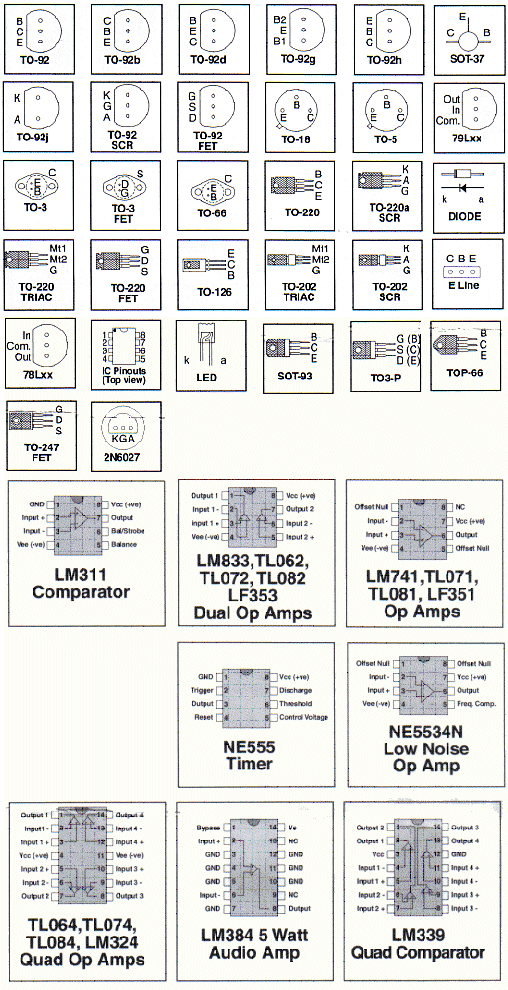Page 75

STEAM SIMULATOR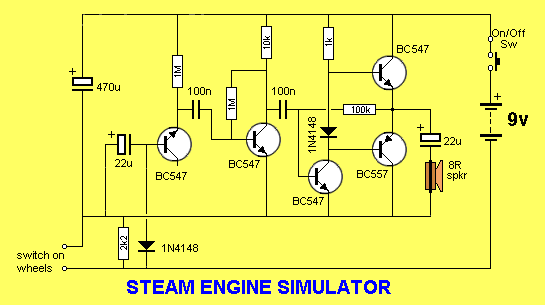A realistic steam sound can be generated with a 4-transistor directly-coupled amplifier connected to a small speaker. The “white noise” is generated by the breakdown across the junction of a transistor and it is activated by a switch made up of contacts touching the wheel of one of the carriages. As the train speeds up and slows down, the sound corresponds to the movement.   See Talking Electronics website for the full project.

Page 76.Here is the circuit from a 27MHz remote control car. It is a simple single-channel link that activates the car in the forward direction when no carrier is being received, and the motor reverses when a carrier is detected. See Talking Electronics website for more details – 27MHz Links.Page 77

This is a single channel receiver, similar to the circuit above.
It can be modified to turn on a “latch” a relay. This means the relay can be turned on remotely but
it cannot be turned off.  The second circuit shows the modification to turn the relay ON with a short
tone and OFF with a long tone.The relay can be turned on but not turned offThe relay can be turned on with a short
tone and turned off with a long tone

SOLAR CHARGER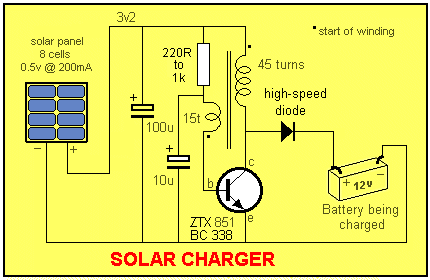This solar charger can be used to charge a 12v battery from any number of solar cells. The circuit automatically adjusts for any input voltage and any output voltage.   See Talking Electronics website for the full project.

Page 78

ARC WELDER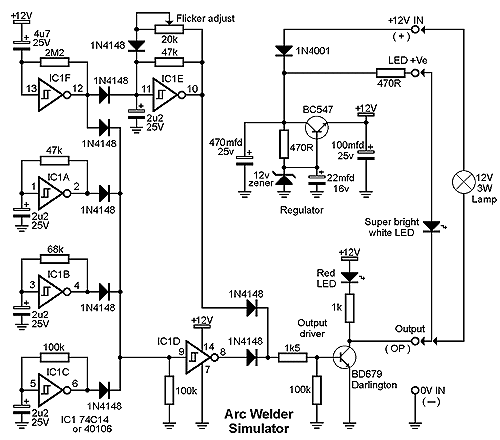The Arc Welder project is one of many projects for model railroads - see Talking Electronics
website for the list of projects.

Page 79

Field strength Meter MkII
.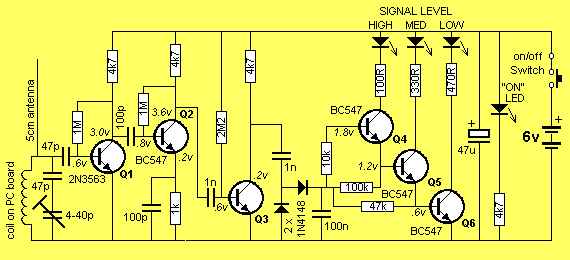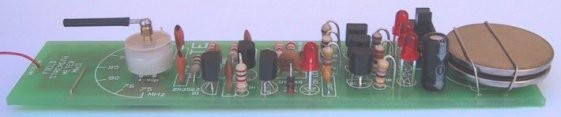A field strength meter is a very handy piece of test equipment to determine the output of a transmitter.
Talking Electronics website describes a number of Test Equipment projects to help with developing your projects.

Page 80

INFINITY BUG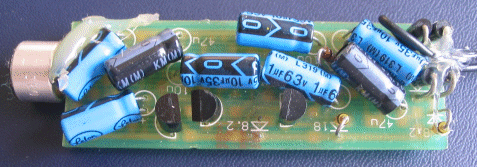THE SURFACE-MOUNT COMPONENTS OF THE INFINITY BUG

The Infinity Bug sits on a remote phone and when the handset is returned to the rest position, the caller whistles down the line and a very sensitive microphone connected to the infinity bug is activated and any audio within 5 metres is detected.

Page 81

FM BUG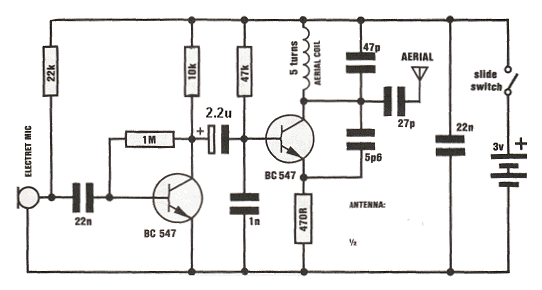FM BUG CIRCUITFM TRANSMITTER   - 88MHz – 108MHz

The FM Bug is one of many FM transmitters designed by Talking Electronics, to show how
far a simple transmitter can reach on a few milliwatts. It is most fascinating to see your
transmitter being detected at 400metres.

3-Transistor Amplifier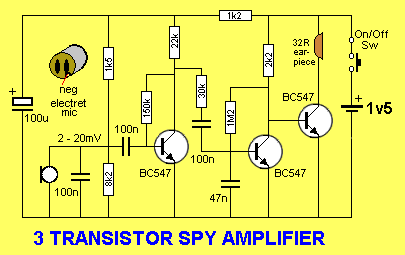The surface-mount 3-Transistor amplifier

HEARING AID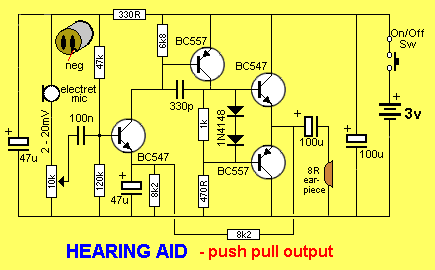Page 83

THE AMMETER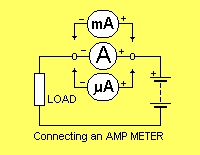(0 - 1uA uses a 1uA movement)THE MICROPHONE
Basically there are two different types. One PRODUCES a voltage and the other REQUIRES a voltage for its operation.
This means you need to supply energy to the second type and this is very important when you are designing a battery-operated circuit and need to have a very low quiescent current.
Here is a list of different types of microphones and their advantages:

 SUPPLY VOLTAGE REQUIRED: Electret Microphone - sometimes called a condenser microphone. Requires about 2-3v @ about 1mA. Extremely good reproduction and sensitivity - an ideal choice. Output - about 10 - 20mV Carbon Microphone - also called a telephone insert or telephone microphone. Requires about 3v - 6v. Produces about 1v waveform. Not very good reproduction. Ok for voice. NO SUPPLY VOLTAGE REQUIRED: Crystal Microphone - also called a Piezo microphone. Produces about 20-30mV Produces a very "tinny" sound - like talking into a tin. Dynamic Microphone - also called a Moving-Coil, Moving-Iron, Magnetic Microphone or Ribbon Microphone. Very good reproduction. Produces about 1mV. A speaker can be used as a microphone - it is called a Dynamic Mic. or Magnetic mic. - output about 1mV

Page 84

If a microphone produces about 20mV under normal conditions, you will need a single stage of amplification. If the microphone produces only 1mV under normal conditions, you will need two stages of amplification.
The circuits below show the first stage of amplification and the way to connect the microphone to the amplifier.Connecting an electret
microphone.The 100n capacitor separates the voltage needed by the microphone (about 1v) from the 0.6v base voltage. A good electret microphone can hear a pin drop at 2 metres. A poor quality electret mic produces crackles in the background like bacon and eggs frying.

The internal construction of an electret microphone
Air enters the electret mic via the top holes and moves the thin mylar sheet. This changes the distribution of the charges on the plastic and the changes is passes down the Gate lead to the FET. The FET amplifies the signal and the result is available on the Drain lead.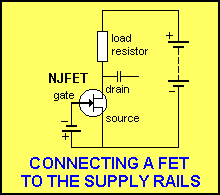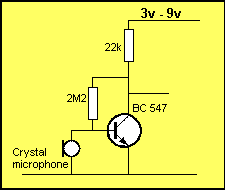Connecting a Crystal  microphone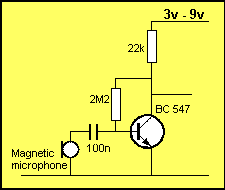The crystal microphone has an almost infinite impedance - that's why it can be connected directly to the base of the transistor.
The magnetic microphone has a very low internal resistance and needs a capacitor to separate it from the base of the amplifying stage. If it is connected directly, it will reduce the base voltage to below 0.7v and the transistor will not operate.

PIEZO DIAPHRAGM
You can also use a piezo diaphragm as a microphone. It produces a very “tinny” sound but it is quite sensitive. Some diaphragms are more sensitive than others, but the sound quality is always terrible.

Page 85

MICROCONTROLLERS

Microcontrollers are the way of the future. Most of the basic theory you will learn for the individual components in this ebook will become very handy when you need to design a circuit.
As a circuit becomes more and more complex, you have a decision to make. Do you want to use lots of individual components or consider using a microcontroller?
Talking Electronics website has a number of projects using individual components and this is the only way the project can be designed. But when it comes to “timing” and requiring an output to produce a HIGH for a particular length of time after an action has taken place, the circuit may require lots of components.

This is where the brilliance of a microcontroller comes in.

It can be programmed to produce and output after a sequence of events and the circuit looks “magic.”  Just one component does all the work and a few other components interface the inputs and output to the chip.

The second special thing about micros is the program.
This has been produced by YOU and it can be protected from “prying eyes” by a feature known as “code protection.”
This gives you exclusive rights to reproduce the project and all your hard work can be rewarded by volume sales.

This is the future.

Talking Electronics website has a number of very simple projects using microcontrollers and these chips all belong to the PIC family of micros.

These chips are very easy to program as they only have 33 - 35 instructions and they can perform amazing things.
See the Talking Electronics website for project using these micros.
The three micros covered on the website are:   PIC12F629, PIC16F84 and PIC16F628.  The MCV08A is a Chinese version of the PIC12F629 and has some extra features and some of the features in the PIC12F629 are not present. But the cost is considerably lower than the PIC12F629. The Chinese get special deals all the time.

Page 86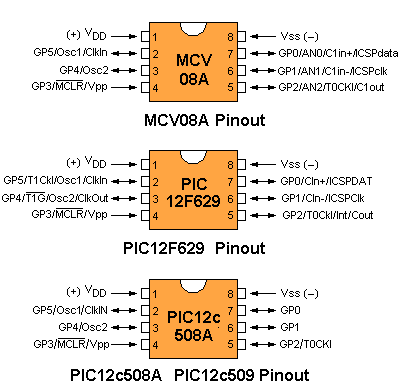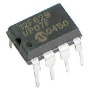HERE IS A PROJECT
USING A MICROCONTROLLER
:

SIMONSIMON PROJECT USING PIC16F628

SIMON is the simple game where you repeat a sequence of flashing coloured lights.
All the “workings” of the project are contained in the program (in the PIC16F628
microcontroller) and the program is provided on Talking Electronics website.
See Simon project for more details.

This completes Data Book 1.   Look out for more e-books on Talking Electronics website:

Sept 2008    Nothing is  copyright.  You can copy anything.       Colin Mitchell

back to pages:    42 to 62